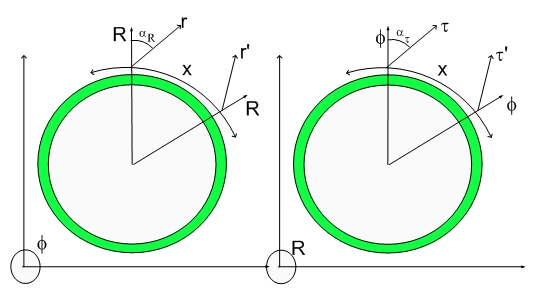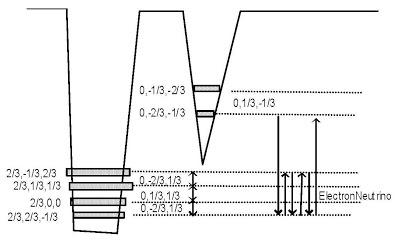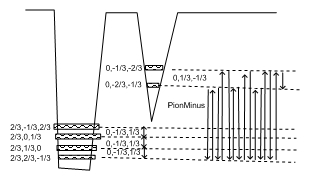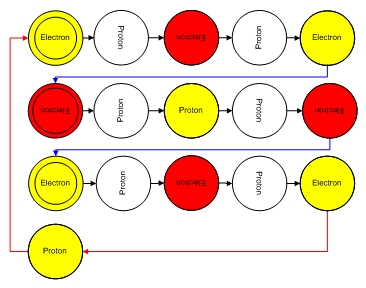# Pion MinusPions and Neutrinos

Their coherence map is given by the four notes. Any spin half particle has always a four notes chord. I don't know anything about music, but I will make the analogy between a 180 degrees tunelling to a musical note. Since 180 degrees tunelling corresponds to 90 degrees spinning and 360 degrees corresponds to four of those events, each particle corresponds to at least four notes.The coherence is between the two Electron states. They differ just by a rotation around x. Axis are given by (0,-2/3,-1/3) and (0,-1/3,-2/3). The boldening of the first axis lenght or quantum number means that spin happens in a direction perpendicular to the x-axis and the radial axis (the fourth dimension). This is important since one has to add up half-spin associated with each chord. The neutrino chord has spin half, similarly to the neutrino particle of the standard model.

It is important to notice that the tunneling frequency between the two Electron states is different from the fundamental dilator frequency and thus the interaction among neutrinos and the rest of the Universe goes in and out of phase as the neutrinos travels, resulting in a vanishingly small interaction. This is why Neutrinos are low interacting particles. The other reason can be understood from the fact that the 4D particle is the rotation of a two dimensional ellipse (with non-zero y and z axes) around the axis perpendicular to the Radial direction and the x direction. This would result in zero 3D projection or zero 3D mass.

The mass is also a function (3D Projection) of the total displacement volume of the dilator. The neutrino having the smallest axis lenghts and being part of the electron potential well, will also have the smallest 3D mass (3D projection of the 4D displacement volume).

Notice that the transmultation notes in the Neutron Model have half-neutrino note in their composition (the note is composed of of the four coherence arrows).

The neutrino model is necessary to be able to understand the pion model. The reason for that is because a pion minus will decay into an electron and a neutrino.

Pion Minus

Below is the amazing Pion Minus chord which is composed of four chords or 16 notes.This complex coherence or chord can also be shown as:I am not a musician, but you can read the chords as an electron chord, followed by a electron-positron transmutation note (half-neutrino chord), followed by a positron-electron transmutation note (another half-neutrino), followed by another electron chord, followed by an electron-proton transmutation note and lastly and proton-electron transmutation note.

The last note recovers the first state of the coherence and completes the Pion Minus Chord.

The reason for the Electron-Proton transmutation notes is because of they do not add charge to the Pion. The charge is just the two electrons and one positron, resulting in a net one negative charge.

The Pion minus decay is envisioned as the break up of this note into two electons, one neutrino and a positron. Positron and electron anihilate transfering their energy to the departing electron.

Things are trickling down as I can. It has been cold and I managed to get a cold myself...:)

I will post next the Pion Plus.

Cheers,

MP

Currently unrated

### New Comment

required

required (not published)

optional

required